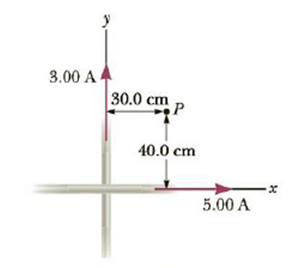Chapter 19, Problem 67AP

Chapter
Section
Textbook Problem

Two long, straight wires cross each other at right angles, as shown in Figure P19.67. (a) Find the direction and magnitude of the magnetic Field at point P, which is in the same plane as the two wires. (b) Find the magnetic field at a point 30.0 cm above the point of intersection (30.0 cm out of the page, toward you).Figure P19.67

a)

To determine
The direction and magnitude of the magnetic field at point P .

Explanation

Given info:

The wire along the x-axis carries current of 5.00A . The current along the y-axis carries current of 3.00A . The distance of the point P from x-axis is 40.0cm , where as from y-axis is 30.0cm .

Explanation:

The arrangement of the wires is shown in the following figure.

The magnetic field of a long straight wire is given by,

B=μ0I2πr

• μ0 is the permeability of free space
• I is the current
• r is the distance of the point from the straight conductor

Consider the positive field to be out of the plane.

The magnetic field at point P due to the wire along x-axis will be out of the page, whereas due to the wire along y-axis it will into the page.

The magnetic field at P due to the wire along x-axis will be,

Bx=μ0Ix2πrx

• Ix is the current in the wire along the x-axis
• rx is the distance of the point P from the x-axis

Bx is positive since it is pointing out of the page.

The magnetic field at P due to the wire along y-axis will be,

By=μ0Iy2πry

• Iy is the current in the wire along the y-axis
• ry is the distance of the point P from the y-axis

By is negative since it is pointing into the page.

The net magnetic field at the point P will be,

Bnet=μ0Ix2πrxμ0Iy2πry=μ02π(IxrxIyry)

Substitute 4π×107TmA-1 for μ0 , 5

b)

To determine
The magnetic field at a point 30.0cm above the point of intersection of the wires.

Still sussing out bartleby?

Check out a sample textbook solution.

See a sample solution

The Solution to Your Study Problems

Bartleby provides explanations to thousands of textbook problems written by our experts, many with advanced degrees!

Get Started

Describe how Dalton obtained relative atomic weights.

General Chemistry - Standalone book (MindTap Course List)

Too little in the diet is associated with osteoporosis. a. vitamin B12 b. protein c. sodium d. niacin

Nutrition: Concepts and Controversies - Standalone book (MindTap Course List)

How many autosomes are present in a body cell of a human being? In a gamete?

Human Heredity: Principles and Issues (MindTap Course List)